Question

A piece of solid lead weighing 34.2 g at a temperature of 315 °C is placed...

A piece of solid lead weighing 34.2 g at a temperature of 315 °C is placed in 342 g of liquid lead at a temperature of 376 °C. After a while, the solid melts and a completely liquid sample remains. Calculate the temperature after thermal equilibrium is reached, assuming no heat loss to the surroundings.

The enthalpy of fusion of solid lead is ΔHfus = 4.77 kJ/mol at its melting point of 328 °C, and the molar heat capacities for solid and liquid lead are Csolid = 26.9 J/mol K and Cliquid = 28.6 J/mol K.

What is the answer in degrees celcius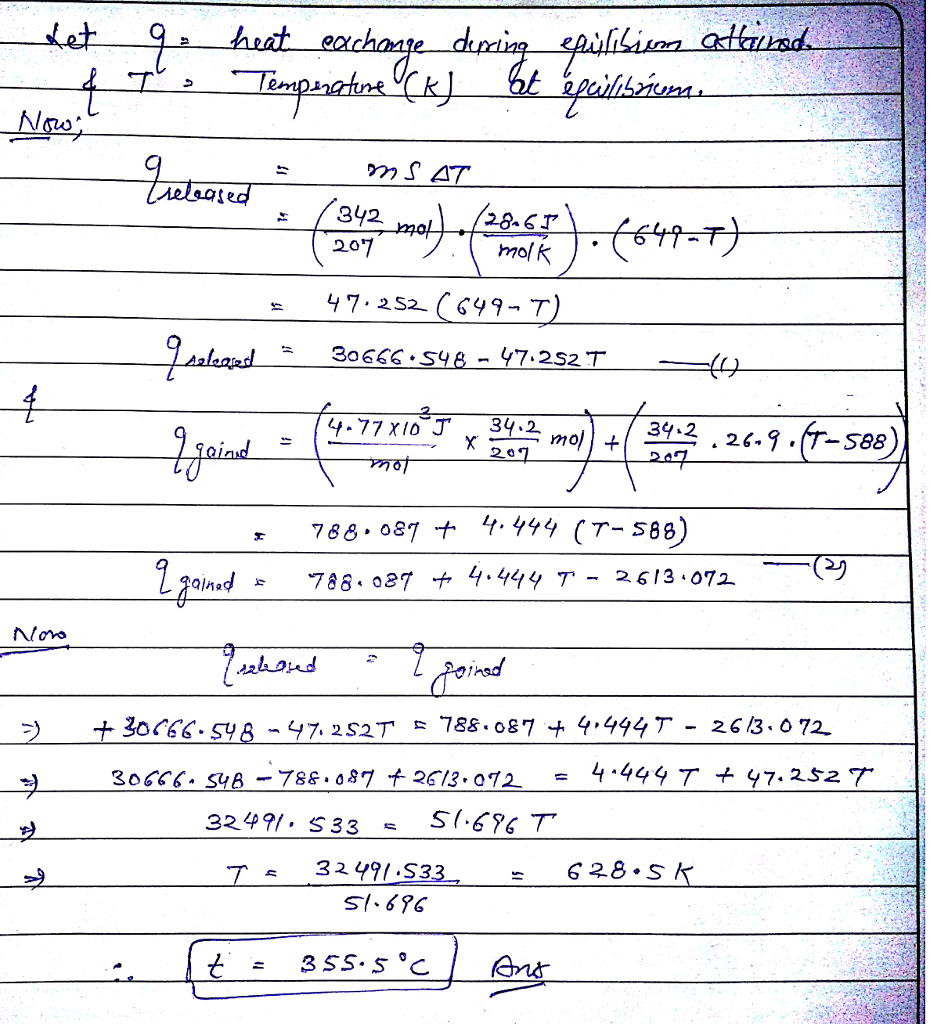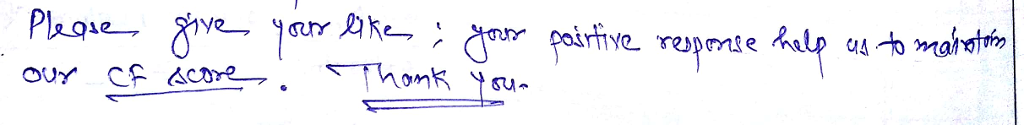Earn Coins

Coins can be redeemed for fabulous gifts.

Similar Homework Help Questions
• A piece of solid lead weighing 32.6 g at a temperature of 311 °C is placed...

A piece of solid lead weighing 32.6 g at a temperature of 311 °C is placed in 326 g of liquid lead at a temperature of 367 °C. After a while, the solid melts and a completely liquid sample remains. Calculate the temperature after thermal equilibrium is reached, assuming no heat loss to the surroundings. The enthalpy of fusion of solid lead is ΔHfus = 4.77 kJ/mol at its melting point of 328 °C, and the molar heat capacities for...

• Determine the amount of heat, in kJ, required to heat 16 g of solid Ca at 851C to 1,375 C. Meltin...

Determine the amount of heat, in kJ, required to heat 16 g of solid Ca at 851C to 1,375 C. Melting Point = 851C Boiling Point = 1487C Molar Heat Capacities: Csolid​ = 26.2 J/mol C C liquid​ = 31.0 J/molC ΔH_fusion​ = 9.33 kJ/mol ΔH_vaporization​ = 162 kJ/mol

• Two 20.0 g ice cubes at -12.0 °C are placed into 215 g of water at...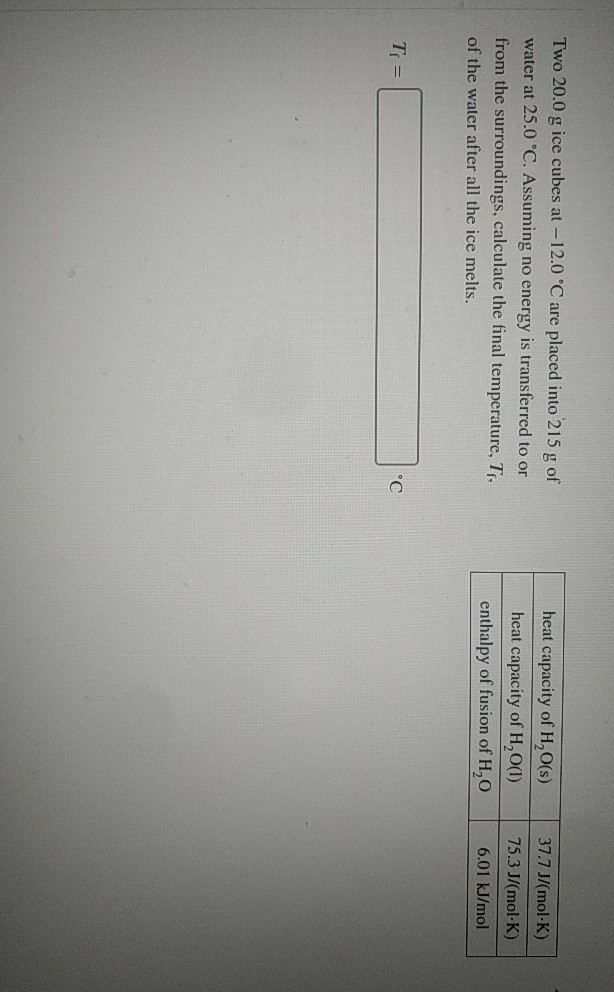Two 20.0 g ice cubes at -12.0 °C are placed into 215 g of water at 25.0 °C. Assuming no energy is transferred to or from the surroundings, calculate the final temperature, T, of the water after all the ice melts. heat capacity of H2O(s) heat capacity of H2O(1) enthalpy of fusion of H,O 37.7 J/(mol-K) 75.3 J/(mol-K) 6.01 kJ/mol Ti =

• A14.92-g block of solid lead at 67.50 °C is immersed in a 23.82-g pool of liquid...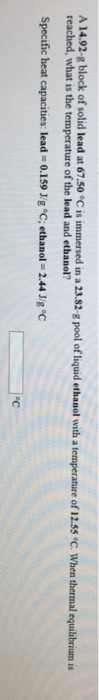A14.92-g block of solid lead at 67.50 °C is immersed in a 23.82-g pool of liquid ethanol with a temperature of 12.55 °C. When thermal equilibrium is reached, what is the temperature of the lead and ethanol? Specific heat capacities: lead - 0.159 Jig °C; ethanol = 2.44 J/g "C

• The following information is given for lead at latm: AH yap(1740 °C) -178 kJ/mol AH fus(328...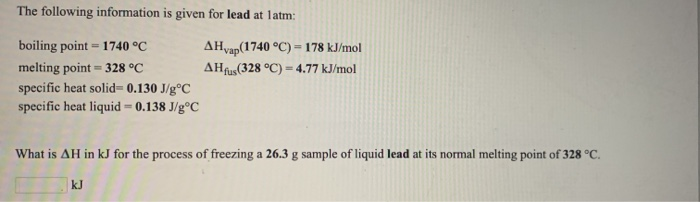The following information is given for lead at latm: AH yap(1740 °C) -178 kJ/mol AH fus(328 °C) = 4.77 kJ/mol boiling point = 1740 °C melting point = 328 °C specific heat solid= 0.130 J/gºC specific heat liquid = 0.138 J/gºC What is AH in kJ for the process of freezing a 26.3 g sample of liquid lead at its normal melting point of 328 °C. The following information is given for silver at latm: AHvap(2212 °C) = 254 kJ/mol...

• The heat of melting for Cu is 13,260 J/mol and the equilibrium melting temperature of Cu...

The heat of melting for Cu is 13,260 J/mol and the equilibrium melting temperature of Cu is 1358 K. Assuming the constant pressure heat capacities of solid Cu and liquid Cu are the same, 24.0 J/(mol K) What is the entropy change for the surroundings, which is also at 1000 K, during solidification from one mole of liquid Cu to one mole solid Cu in the system at 1000 K?

• Two 20.0 g ice cubes at -12.0 °C are placed into 215 g of water at...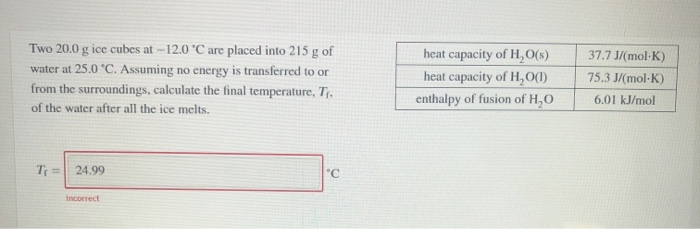Two 20.0 g ice cubes at -12.0 °C are placed into 215 g of water at 25.0 °C. Assuming no energy is transferred to or from the surroundings, calculate the final temperature, T. of the water after all the ice melts. heat capacity of H,O() heat capacity of H,O(1) enthalpy of fusion of H,0 37.7J/(mol-K) 75.3 J/(mol-K) 6.01 kJ/mol Ti = 24.99 "C Incorrect

• 2. 44.0 g of ice at –20.0 °C is mixed with 325 g of water at...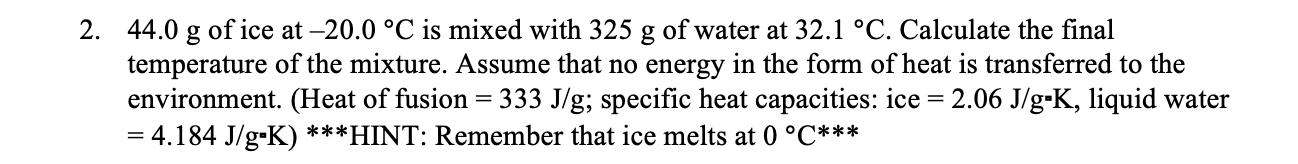2. 44.0 g of ice at –20.0 °C is mixed with 325 g of water at 32.1 °C. Calculate the final temperature of the mixture. Assume that no energy in the form of heat is transferred to the environment. (Heat of fusion = 333 J/g; specific heat capacities: ice = 2.06 J/g-K, liquid water = 4.184 J/g-K) ***HINT: Remember that ice melts at 0 °C***

• by Two 20 0g ice cubes at-13.0 ℃ are placed into 205 g of water at...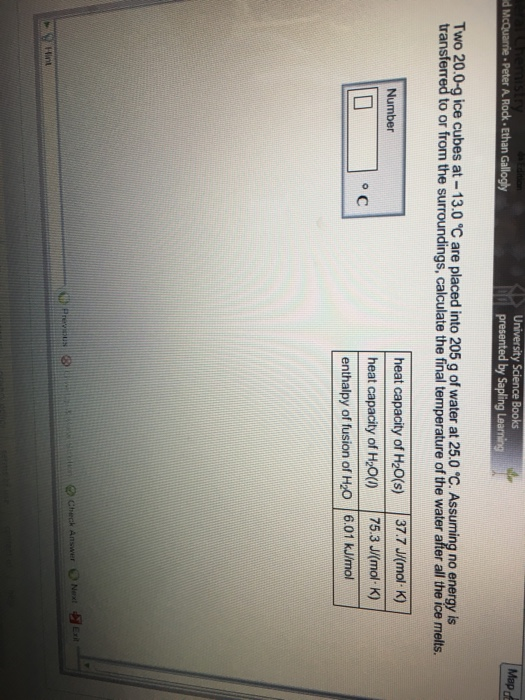by Two 20 0g ice cubes at-13.0 ℃ are placed into 205 g of water at 25.0 ℃ Assuming no transferred to or from the surroundings, calculate the final temperature of the water after all the ice melts heat capacity of H20(s) 37.7J(mol K) heat capacity of H20() 75.3 J(mol K) enthalpy of fusion of H2O 6.01 kJ/mol

• Given the following information for an unknown chemical: Specific heat (c) of solid phase: 1.75 J/g°C Heat of fusion (ΔH...

Given the following information for an unknown chemical: Specific heat (c) of solid phase: 1.75 J/g°C Heat of fusion (ΔHfus): 32.0 kJ/mol Specific heat (c) of liquid phase: 4.50 J/g°C Heat of vaporization (ΔHvap): 400. kJ/mol Specific heat (c) of vapor phase: 1.50 J/g°C Molar mass: 72.5 g/mol Freezing point: -15.00°C Boiling point: 15.00°C 1.Calculate the total energy needed (in kJ) to convert 15.5g of this chemical from -21.5°C to room temperature (23.0°C).